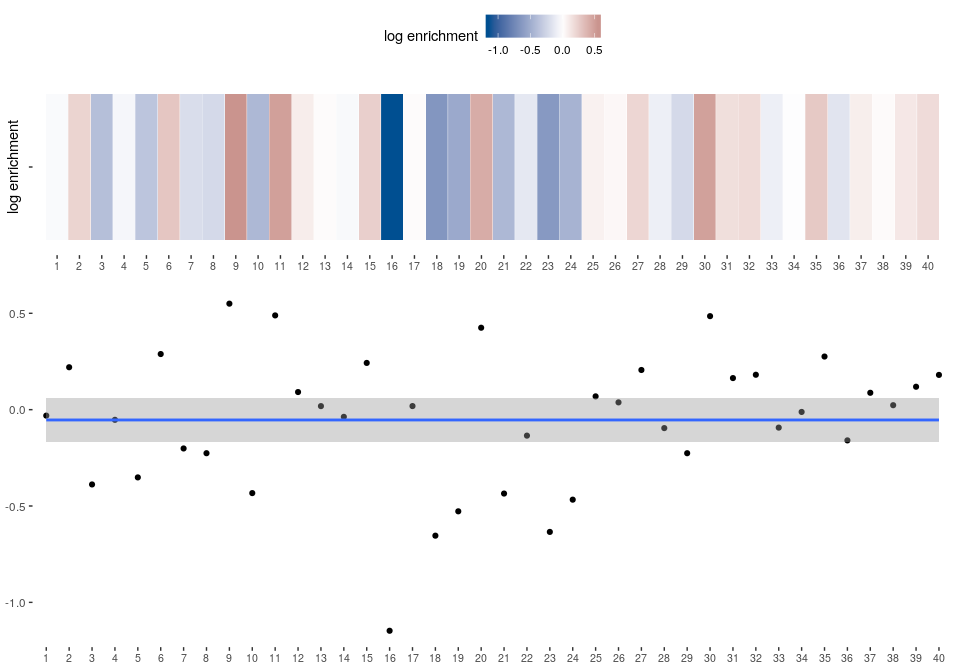# Spectrum Motif Analysis (SPMA)

#### 2019-10-07

In order to investigate how RBP targets are distributed across a spectrum of transcripts (e.g., all transcripts of a platform, ordered by fold change), Spectrum Motif Analysis visualizes the distribution of putative RBP binding sites across all transcripts.

# Analysis

library(transite)

Load example data set from transite package, a data frame with 1000 rows and the following columns:

• refseq: RefSeq identifiers
• value: signal-to-noise ratios between treatment and control samples (sorting criterion)
• seq.type: specifying the type of sequence in seq column; either 3’-UTR, 5’-UTR, or entire mRNAs (including 5’-UTRs, coding regions, and 3’-UTRs)
• seq: sequence
background.df <- transite:::ge$background Sort sequences (i.e., transcripts) by ascending signal-to-noise ratio. Transcripts upregulated in control samples are at the beginning of list, transcripts upregulated in treatment samples at the end. background.df <- dplyr::arrange(background.df, value) The DNA sequences in the data frame are converted to RNA sequences. Motifs in the Transite motif database are RNA-based. background.set <- gsub("T", "U", background.df$seq)

Prepare named character vector of presorted sequences for runMatrixSPMA. The function expects a character vector containing sequences, sorted in a meaningful way, named according to the following format: [REFSEQ]|[SEQ.TYPE]

The name is only important if results are cached. It is used as the key in a dictionary that stores the number of putative binding sites for each RefSeq identifier and sequence type.

names(background.set) <- paste0(background.df$refseq, "|", background.df$seq.type)

In this example we limit our analysis to an arbitrarily selected motif in the motif database in order to reduce run-time.

motif.db <- getMotifById("M178_0.6")

Matrix-based SPMA is executed:

results <- runMatrixSPMA(background.set, motifs = motif.db, cache = FALSE)

# Usually, all motifs are included in the analysis and results are cached to make subsequent analyses more efficient.
# results <- runMatrixSPMA(background.set)

# Results

Matrix-based SPMA returns a number of result objects, combined in a list with the following components:

• foreground.scores
• background.scores
• enrichment.dfs
• spectrum.info.df
• spectrum.plots
• classifier.scores

In the following, we plot the spectrum plot, showing how putative binding sites are distributed across the spectrum of transcripts. (spectrum.plots).

In addition, we show statistics describing the spectrum plot (spectrum.info.df).

####BRUNOL6, CELF3 (M178_0.6)

Spectrum plot with polynomial regression:Classification:

spectrum classification: random (0 out of 3 criteria met)

Property Value Threshold
adjusted $$R^2$$ 0 $$\geq 0.4$$
polynomial degree 0 $$\geq 1$$
slope 0 $$\neq 0$$
unadjusted p-value estimate of consistency score 0.9426115 $$< 0.000005$$
number of significant bins 0 $$\geq 4$$

Visual inspection of the spectrum plot as well as the classification and the underlying properties suggest that the putative binding sites of this particular RNA-binding protein are distributed in a random fashion across the spectrum of transcripts. There is no evidence that this RNA-binding protein is involved in modulating gene expression changes between treatment and control group.